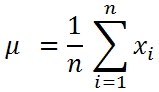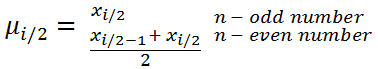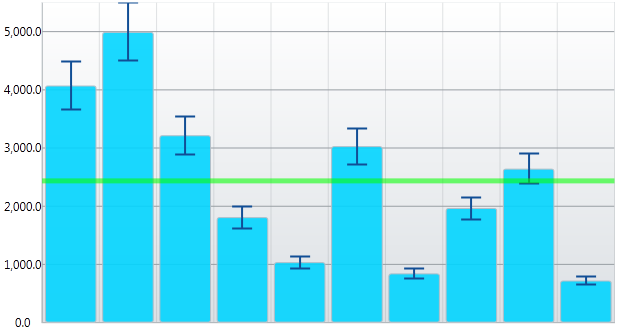# How to use the Infragistics Math Calculators

Damyan Petev / Friday, November 18, 2011

In a previous post we looked into using XamDataChart along with a Correlation calculator. But there’s much more – the Infragistics Math Calculators™ library offers quite a few calculators that can be used to perform mathematical or statistical calculation. Their main focus is as calculators for Value Overlay and Data Correlation or Error Bars for series in the XamDataChart or even as stand-alone for most of the value based ones. Mentioning Error Bars - there’s actually a whole post on the subject you can find following this link. The calculators can be classified in two groups based on how they function.

The first group would be the value calculators – they compute and expose a single value. While their names are quite self-explanatory a line or two for clarification is in order. The Infragistics Math Calculators™ library contains the following value calculators:

• Correlation and CorrelationCalculator mostly covered by a post already. All that can be added is the formula – but it’s complicated and long enough to just skip it. You can always visit our documentation and have a look.
• The MeanCalculator uses the mathematical method to get the central tendency of some data often referred as simply mean or average.Where µ is the mean, xi is the variable at position ‘i’, and n is the total number of members of the data. This is however prone to value spikes caused by a few very large or very small variables the result of which is not always what one would perceive as average.

• MedianCalculator also measures central tendency but is the more robust method when it comes to dealing with outliers. What it does is sort the data and takes the variable separating the higher values part from the lower one. Or if there are two separating values takes their average. Using the same naming as above its formula would look like:• Standard Deviation computed by the StandardDeviationCalculator is a measure of how close or how far the data points are positioned around their mean. What this means is that the larger the deviation the wider is the range of values spreading. Besides expressing the dispersion of the data it is also used as confidence measure for conclusions based on statistics.
• Standard Error (StandardErrorCalculator) in sample statistics is the standard deviation of sampling distribution of a statistic. In our case calculator will provide result based on the mean (standard error of the mean).

Formulas for those can be found in our documentation as well as in the blog post linked above about Error Bars.

• VarianceCalculator computes the variance which is a measure of how far a set of values are spread out from each other, in this case from the mean. It is equal to the square of the standard deviation.

The other group of calculators is those supported by the XamDataChart Error Bars. Some are more closely related than others.

• The DataCalculator takes a series of pre-calculated error values and applies them as Error Bar for the respective values.
• The FixedValueCalculator takes a single static value to be used on all.
• The PercentageCalculator takes a value between 0 and 100 based on which it will calculate the percentage for each value.
• The StandardDeviationCalculator and StandardErrorCalculator described above can also be used as calculators for the Error Bars.

## Requirements

Almost all calculators require the data model to implement the IEnumerable interface (e.g. Lists, Collections and so on) and contain at least one column with numeric data for calculation. Since the calculators can be used with multiple NetAdvantage products the assembly references required will vary based on the platform – WPF, Silverlight, Windows Phone and so on. Generally they are the main Infragistics assembly, the Math and the Math Calculators (e.g. InfragisticsWPF4.v11.2.dll, InfragisticsWPF4.Math.v11.2.dll, InfragisticsWPF4.Math.Calculators.v11.2.dll).

## Using the calculators

The calculators use their ItemSource property for binding and their ValueMemberPath for mapping. Setting up the calculator is quite simple and can be done in XAML:

1. xmlns:ig="http://schemas.infragistics.com/xaml"
1. <Grid>
2.     <Grid.Resources>
3.         <!--Mean Calculator-->
4.         <ig:MeanCalculator x:Key="meanCalc" ItemsSource="{StaticResource data}" ValueMemberPath="Value" />
5.     </Grid.Resources>
6.     <!--Display Results-->
7.     <TextBlock Text="{Binding Value,Source={StaticResource meanCalc}}" />
8. </Grid>

Or in C# or Visual Basic:

1. using Infragistics.Math.Calculators;
1. DataPointSample data = new DataPointSample();
2. MeanCalculator meanCalculator = new MeanCalculator();
3. meanCalculator.ItemsSource = data;
4. meanCalculator.ValueMemberPath = "Value";
5. double result = meanCalculator.Value;

In these examples the calculator is used as stand-alone component and as it lacks visual representation it is usually defined as resource in an element or the whole application depending on your needs. The code is very similar for all so you can replace the Mean Calculator with different one but there are exceptions to the rule:

• The Correlation Calculator as you might have seen in the post about it takes two rather than one column of numeric values so naturally it has two ValueMemeberPath properties to set for them.
• The Fixed Value Calculator and the Percentage Calculator take a single Value so they lack the Item Source and the member Path properties. They are also tightly coupled with the XamDataChart and serve little to no purpose outside. The Percentage Calculator uses it’s value along with the variables for the chart to calculate the length of the Error Bar and the Fixed Calculator used to set a single Value would be nothing but a constant on its own.

## Using with XamDataChart

There are three main usages for the calculators when working with the XamDataChart and two have already been mentioned and covered – calculating data correlation and adding Error Bars.

Correlation calculator is always defined within a resources section unless in code behind and looks like:

1. <Window.Resources>
2.     <ig:CorrelationCalculator
3.         x:Key="correlation"
4.         XMemberPath="X"
5.         YMemberPath="Y"
6.         ItemsSource="{StaticResource data}"/>
7. </Window.Resources>

Error Bars are defined within series in the XamDataChart and they can be vertical and/or horizontal depending on the type the specific series support and an example of how to define them can look like:

1. <ig:ColumnSeries.ErrorBarSettings>
2.     <ig:CategoryErrorBarSettings StrokeThickness="2" Stroke="#FF114E6C"
3.                                  EnableErrorBars="Both" ErrorBarCapLength="20">
4.         <ig:CategoryErrorBarSettings.Calculator>
5.             <ig:PercentageCalculator Value="10"/>
6.         </ig:CategoryErrorBarSettings.Calculator>
7.     </ig:CategoryErrorBarSettings>
8. </ig:ColumnSeries.ErrorBarSettings>

The third usage is to calculate Value Overlay which is used to overlay a single value as a line over the series to indicate some important points such as the mean. It will be shaped as a line when bound to Numeric Axis and a circle when bound to Angle or Radius Axis. It is defined much like other series but rather than taking a source of data it needs a single Value to be set.

1. <ig:ValueOverlay Axis="{Binding ElementName=numericYAxis}"
2.      Value="{Binding Value,Source={StaticResource meanCalc}}"
3.      Thickness="5" Brush="#FFF59635">
4. </ig:ValueOverlay>

And this is how a XamDataChart can look using Column series with Error Bars calculated as 10% of each and a the mean value marked with Value Overlay:## Custom Calculators

Further customization is available by creating a new calculator to perform specific functions and meet all requirements. This can be done by inheriting from the base ValueCalculator class. It provides the ItemSource to bind data to and calculate a Value. Logic can be provided to override the Calculate method:

1. //Define a Custom Calculator
2. /// <summary>
3. /// Calculates the Index of dispersion of set of data.
4. /// </summary>
5. /// <param name="input"> The input list of double values.</param>
6. public class IndexDispersionCalculator : ValueCalculator
7. {
8.     public override double Calculate(System.Collections.Generic.IList<double> input)
9.     {
10.         VarianceCalculator _variance = new VarianceCalculator();
11.         MeanCalculator _mean = new MeanCalculator();
12.         double result;
13.         if (_mean.Calculate(input) == double.NaN)
14.         {
15.             return double.NaN;
16.         }
17.         else
18.         {
19.             result = _variance.Calculate(input) / _mean.Calculate(input);
20.         }
21.         return result;
22.     }
23. }

As can be seen this is a calculator that will compute the Index of dispersion or variance-to-mean ratio (VMR) also known as Fano factor. While names sound all complicated it really is the ratio between the variance and the mean and since we have Calculators for those already we can just use their Calculate methods to get values for our new one. Of course the requirements will dictate the logic for this and it be anything you can think of and implement – from minimum/maximum possible value to complicated calculations.

Once you have you calculator you can define it in XAML and use it pretty much like any other:

1. xmlns:local="clr-namespace:MathCalculatorsDemo"
1. <!--Somewhere inside a resource section-->
2. <!--Custom Calculator for dispersion index-->
3. <local:IndexDispersionCalculator x:Key="disperIndexCalc" ItemsSource="{StaticResource data}" ValueMemberPath="Value"/>

## Conclusion

The Infragistics Math Calculators™ library provides powerful calculation tools in the form of various mathematical and statistical calculators and close integration with the XamDataChart with Error Bars, Value Overlay and Data Correlation calculations. The library will not only save time and support valuable functionality for the chart but will also offer the chance to create and use custom calculators with great ease.

More information and examples for each can be found in our documentation

And a demo project demonstrating some of the features in WPF project (the XAML and code behind you can easily take and use in Silverlight too) can be downloaded from here.